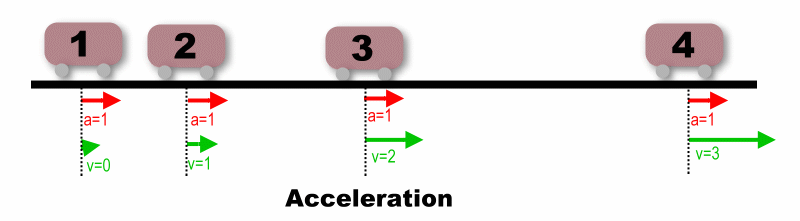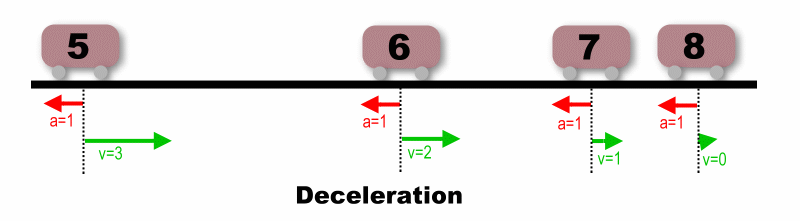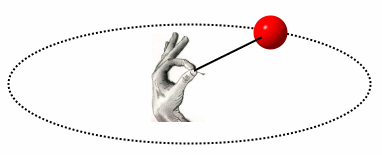# Physical Background

Without trying to scare anybody off, there's a little physics that you ought to know in order to get some intuition for the design principles in the rest of these notes. A few underlying physical principles are covered in this chapter. I've tried to make them as intuitive and painless as possible. I strongly recommend you try to understand it, though the rest of the notes aren't a total loss even if you don't.

The topics covered here are:

## Force, Acceleration, and Velocity

Physics is studied at three different levels:
1. Tiny, as in nuclear physics and quantum physics.
2. Huge, as in astrophysics.
3. In between, on a scale that we experience every day.
When you hear names like Einstein, you're in the realm of the first two levels. But the physics that is interesting in golf exists at the third level, and most of what we know there started with Isaac Newton. The physics of golf is a niche of "Newtonian physics", and specifically part of Newtonian dynamics -- the study of moving objects. So let's review some of the very basic tenets of what Newton passed down to us.

First, and most basic: A body in motion remains in motion at the same speed and in the same direction, unless acted upon by a force. (The famous quote, "A body at rest remains at rest" is just a special case of this law; the motion in that case is zero.)

Getting mathematical about it, we've all seen Newton's basic law of motion:
F = ma
That means that, in order to accelerate an object, you must apply a force "F" equal to the mass of the object "m" times the rate at which you want to accelerate it "a". Many of the interesting questions in golf depend on this equation.

But what is acceleration? It's the rate at which velocity changes. ("Velocity" is a speed with a direction. For instance, 100mph is a speed. 100mph to the north with an upward angle of 3 degrees is a velocity.) Example:
• An object is moving at 10 miles per hour.
• Once second later, that object is moving in the same direction at 13 miles per hour.
• So the acceleration of that object is 3 miles per hour per second. (Acceleration is measured as speed difference per unit of time.)
Here's another aspect of acceleration. If you listen to a teaching pro or a TV golf announcer, you've heard that deceleration is the opposite of acceleration. That's sort of right, but not exactly. Deceleration is acceleration, but in the direction opposite to velocity.

Acceleration opposite to velocity? What's going on here? Here's an example to illustrate it.Here is a movable mass. We've put it on wheels so there is no friction; any force on the mass goes straight into acceleration, with nothing drained off to overcome friction. Let's see what happens when we apply a constant force to the right, just enough force to give an acceleration of one mile per hour per second. The numbered stations are positions of the mass at one-second intervals. Here's what's going on at each of the numbered stations.
1. The mass is at rest. Velocity "v" is zero. We apply a force to the right, just enough to give an acceleration of 1 mph per sec. The mass begins to move to the right.
2. After the first second, the velocity is 1mph -- that's what an acceleration of 1mph per second means. The velocity is to the right, the same direction as the force producing the acceleration.
3. Another second, and the velocity is now 2mph to the right. No big surprise here.
4. Yet another second; the mass is moving farther and faster, and is now up to 3mph to the right.
Now the mass is clipping along to the right at 3mph. If we remove the force and just let it roll, it will keep rolling to the right at 3mph "forever" -- or at least until friction slows it down. That's what Newton says.

But now let's apply the same force we had before, to the same object which is now moving at 3mph to the right. But we apply a force to the left. This gives an acceleration to the left of 1mph per second.1. This is the moment at which we start to apply the force. The velocity is 3mph to the right, but the acceleration is 1mph per second to the left.
2. After the first second, the leftward acceleration has removed 1mph from the rightward velocity. That is deceleration: a reduction of velocity by adding an acceleration in the opposited direction from the velocity.
3. Another second, and the velocity is now down to 1mph to the right.
4. Now the mass has stopped -- zero velocity -- because the leftward acceleration has completely "decelerated" the rightward velocity.
So a force in the same direction as velocity will create an acceleration to increase the velocity, and a force in the opposite direction as velocity produces an acceleration to decrease the velocity.

## Centrifugal Force

Now we know about acceleration in the same direction as velocity and acceleration in the opposite direction to velocity. But what if we apply a force outside the direction of velocity -- say, at right angles to velocity? What does acceleration in that direction do?Have you ever twirled a ball on a string? If not, try it. (It doesn't have to be a ball, just something with several ounces of mass to it.) You will feel a force; you have to pull on the string to make it work. The faster you twirl the ball, the more force you will exert on the string. And the heavier the ball, the more force you will exert on the string. The phenomena at work here are called "centripetal force" and "centrifugal force". Here's what is going on.Remember Newton? What would he say? In the absence of a force, the ball would move in a straight line -- a constant velocity to the left. In the picture, that is labeled "Path without force". In order to make the ball move in a circle, we need to exert a force on the ball, in the direction of the curvature we want to make in the ball's path. A force on a moving ball at right angles to the direction the ball is moving has no component either helping or opposing the existing velocity. So it will not change the speed of the ball, but it will change the direction. Remember, velocity is a combination of speed and direction. In this case, acceleration does change the velocity, but only the direction component. It will put a curve on the ball's path. Such a curve-producing force is called "centripetal force". When we twirl a ball on a string, we are exerting a centripetal force on the ball through the string. Why do we feel a pull on the string? Let's turn to Newton again. He also pointed out that, for every force, there is an equal and opposite reaction force. So we are feeling the pull on the string in reaction to the centripetal force we put on the ball. The reaction force to a centripetal force is called a "centrifugal force", and it is equal to the centripetal force and opposite in direction. Here are some properties of centripetal and centrifugal force: The faster you twirl the ball, the stronger the force. The heavier the ball, the stronger the force. The tighter the curvature you want, the stronger the force has to be. (A "tight curvature" means a smaller radius to the circle you're twirling.) As an equation, it looks like:      m v2 F = ------       r Where: F = the centripetal or centrifugal force. m = the mass of the moving body. v = the velocity (really just the speed this time) of the moving body. r = the radius of the circle you want the body to travel, called the "radius of curvature". There is some debate over whether centrifugal force is real. Indeed, some is and some isn't. But we aren't going to worry about it. For the limited purposes of explaining the motion of a golf club, we don't have to split hairs; the explanations and calculations work whether we treat the force as real or compute it as an acceleration. For the time being, it is the simplest way I know to explain the golf swing to a beginner -- at physics, not golf. There are other ways to explain it that are closer to the way a scientist would look at it, and I hope to do an article on that sometime. But it isn't here yet.